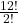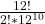## We assume each month is equally likely to be a student’s birthday month. i) The number of ways ten students can have birthdays in 10 differe

Question

We assume each month is equally likely to be a student’s birthday month. i) The number of ways ten students can have birthdays in 10 different months is: 12 · 11 · 10 . . . · 3 = 12!/2! ii) The number of ways 10 students can have birthday months is: 1210 iii) The probability that no two share a birthday month is: 12!/(2!.1210) = 0.00387 Is the solution showed correct?

in progress 0
7 months 2021-08-03T00:54:15+00:00 1 Answers 6 views 0

## Answers ( )

The solutions are correct

Step-by-step explanation:

i) In a year there is a total of 12 months, if ten students can have birthdays in 10 different months, the number of ways this can happen is:

Number of ways = 12 × 11 × 10 × . . . × 3 =ii) The number of ways 1 student can have birthday months = 12¹, The number of ways 2 students can have birthday months = 12¹ × 12¹ = 12². Hence:

The number of ways 10 students can have birthday months = 12¹⁰

iii) The probability that no two share a birthday month == 0.00387